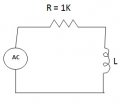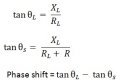# Doubt on RL circuit

#### simpsonss

Joined Jul 8, 2008
173
Hi,
Below is my RL circuit which resistor and inductor are in series and the output waveform is across the inductor.I'm referring to the phasor diagram to calculate the phase shift value between input and output. The formula i figured out is as below:Am i in the correct way?

#### Georacer

Joined Nov 25, 2009
5,182
Can you specify across which component is the output voltage measured? It is significant.
Why have you assumed that the coil has resistance? Is it mentioned somewhere?

I also assume that L is for load and S is for source.

#### Georacer

Joined Nov 25, 2009
5,182
Ok, assuming that the output is across the coil, consider this:

$$V_{out}=I_L \cdot Z_L \\ =\frac{V_S}{Z_{total}} \cdot Z_L$$

The angle of that phasor is the phase shift, which, of course, is dependant on the source frequency.

(is it dependant or dependand? Which is for persons and which is the adjective?)

#### djsfantasi

Joined Apr 11, 2010
9,131
Aside:
(is it dependant or dependand? Which is for persons and which is the adjective?)
"Your dependant is dependent upon you."

"Dependent" is the adjective, meaning to be reliant upon. "Dependant" is the noun.

•Georacer